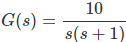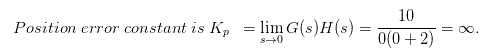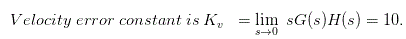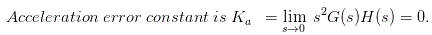# MCQs on Control Systems

##### Page 11 of 23. Go to page 1 2 3 4 5 6 7 8 9 10 11 12 13 14 15 16 17 18 19 20 21 22 23
01․ When the polynomial is Routh Hurwitz,
the function is not real.
the roots of function have real parts which are to be zero/negative.
all zeroes lie in the right half of the s-plane.
none of these.

When the polynomial is Hurwitz then the roots of the function have real parts which are to be zero / negative.

02․ Time response for a second order system depends on the value of τ. If τ= 0 then the system is called as
undamped system.
under damped system.
critically damped system.
over damped system.

The damping ratio is a dimensionless measure describing how oscillations in a system decay after a disturbance. Many systems exhibit oscillatory behavior when they are disturbed from their position of static equilibrium. If τ is zero then there will be no damping, hence, it is called undamped system. Where the spring mass system is completely lossless, the mass would oscillate indefinitely, with each bounce of equal height to the last. This hypothetical case is called undamped.

03․ Time response for a second order control system depends on value of ξ. If ξ>1 then the system is called as
undamped system .
under damped system.
over damped system.
critically damped system.

Commonly, the mass tends to overshoot its starting position, and then return, overshooting again. With each overshoot, some energy in the system is dissipated, and the oscillations die towards zero. This case is called over damped. For over damped system ξ is greater than 1.

04․ Time response for a second order system depends on value of ξ. If ξ = 1 then the system is called as
undamped system.
under damped system.
over damped system.
critically damped system.

Between the over damped and under damped cases, there exists a certain level of damping at which the system will just fail to overshoot and will not make a single oscillation. This case is called critical damping.

05․ Time response for a second order system depends on value of ξ. If ξ < 1 then the system is called as
under damped system.
undamped system.
over damped system.
critical damped system.

If the system contained high losses, for example if the spring mass experiment were conducted in a viscous fluid, the mass could slowly return to its rest position without ever overshooting. This case is called under damped. For under damped system ξ is less than 1.

06․ For a unity feedback control system open loop transfer functionthen position error constant is
0.
20.
infinity.
40.07․ For a unity feedback control system open loop transfer functionthen velocity error constant is
10.
50.
infinity.
0.08․ For a unity feedback control system open loop transfer functionthen acceleration error constant is
0.
50.
infinity.
20.09․ For a transfer function H (s) = P(s) / Q(s), where P (s) and Q (s) are polynomials in s. Then
the degree of P (s) is always greater than the degree of Q (s).
the degree of P (s) and Q (s) are same.
degree of P (s) is independent of degree of Q (s).
maximum degree of P (s) and Q (s) differ at most by one.

If the degree of the numerator P (s) and the denominator Q (s) are dependent with each other, then the poles and zeros of the system may gets placed on the same roots. Then the transfer function is wrong. So, the degree of the numerator P (s) and the denominator Q (s) should be independent with each other. This is the one of the necessary conditions for transfer function.

the system parameters alone.
nature of the input.
initial conditions of input and output.
nature of the output.

The transfer function is nothing but the ratio to the Laplace transform of the output to the Laplace transform of the input. So, based on the input and output characteristics the system characteristics can be changed. But, if we adjust the system parameter, the output can change with respect to the input of the system. Hence, due to this action, the transfer function also can be changed. Thus, the transfer function of the control system depends on the parameters of the system alone.

<<<910111213>>>